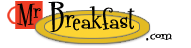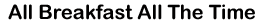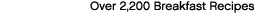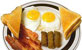Site Index/Glossary > Measurement Conversion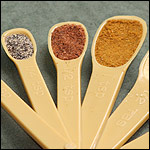# Measurement Conversion

Tablespoon/Teaspoon Conversion

3 teaspoons = 1 Tablespoon
1/2 Tablespoon = 1 and 1/2 teaspoons
1 Tablespoon = 3 teaspoons
1.2 teaspoon (US) = 1 teaspoon (UK)
1.2 Tablespoon (US) = 1 Tablespoon (UK)

Tablespoon/Cup Conversion

4 Tablespoons = 1/4 cup
5 and 1/3 Tablespoons = 1/3 cup
8 Tablespoons = 1/2 cup
10 and 2/3 Tablespoons = 2/3 cup
12 Tablespoons = 3/4 cup
16 Tablespoons = 1 cup
1/8 cup = 2 Tablespoons
1/4 cup = 4 Tablespoons
1/3 cup = 5 Tablespoons plus 1 teaspoon
1/2 cup = 8 Tablespoons
1 cup = 16 Tablespoons

Ounce Conversion

1 teaspoon = 1/6 ounce
1 Tablespoon = 0.5 ounce
2 Tablespoons = 1 fluid ounce
8 Tablespoons = 4 fluid ounces
16 Tablespoons = 8 fluid ounces
1/4 cup = 2 fluid ounces
1 cup = 8 fluid ounces

 Pint/Quart Conversion 1 cup = 1/2 pint 2 cups = 1 pint 2 pints = 1 quart 4 quarts (liquid) = 1 gallonBushel/Peck Conversion 8 quarts (dry) = 1 peck 4 pecks (dry) = 1 bushel
 Metric Conversion - Volume 1/4 cup = 60 milliliters 1/2 cup = 120 milliliters 1 cup = 230 milliliters 1 and 1/4 cups = 300 milliliters 1 and 1/2 cups = 360 milliliters 2 cups = 460 milliliters 2 and 1/2 cups = 600 milliliters 3 cups = 700 milliliters 4 cups = (1 quart) .95 liter 1.06 quarts = 1 liter 4 quarts (1 gallon) = 3.8 liters 50 milliliters = .21 cup 100 milliliters = .42 cup 150 milliliters = .63 cup 200 milliliters = .84 cup 250 milliliters = 1.06 cups 1 liter = approximately 4 cups or 1 quartMetric Conversion - Weight 1/4 ounce = 7 grams 1/2 ounce = 14 grams 1 ounce = 28 grams 1 and 1/4 ounces = 35 grams 1 and 1/2 ounces = 40 grams 2 and 1/2 ounces = 70 grams 4 ounces = 112 grams 5 ounces = 140 grams 8 ounces = 228 grams 10 ounces = 280 grams 15 ounces = 425 grams 16 ounces (1 pound) = 454 grams 1 gram = .035 ounce 50 grams = 1.75 ounces 100 grams = 3.5 ounces 250 grams = 8.75 ounces 500 grams = approximately 1 pound 1 kilogram = approximately 2 pounds